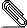# sub foo (\$x) returns ref(\$x)

```Suppose we have a function that takes an argument and returns something
with the same type as that argument.  One previous suggestion is this:```
```
sub identity ((::a) \$x) returns ::a { return(...) }

This is fine if both invariants in the "the meaning of 'returns'" thread
are observed, since the inner &return will check that (...).does(::a),
and the outer context will demand ::a.does(ref(\$x)).

However, this relies on the fact that we unify argument types first,
then use the bounded type variables to handle returns types.  Under that
scheme, It is less clear how to talk about how the types of two arguments
must relate.  For example, assuming argument types are unified in a single
phase, the example below does nothing useful:

sub equitype ((::a) \$x, (::a) \$y) { ... }

It won't not help even if we replace the implicit "does" with "of":

sub equitype (\$x of (::a), \$y of (::a)) { ... }

The reason, in Luke Palmer's words:

"The trouble with this is that it doesn't provide any type safety.
In the case of a type conflict, "a" just degenerates to the Any type."
(cf. pugs/docs/notes/recursive_polymorphism_and_type)

This fact, coupled with the unappealing (::a) syntax, leads me to look
for a more Perlish representation.  Adopting an idea from Odersky's
"Nested Types" paper, we can let terms occur at type variant position,
by introducing a "ref" form for types:

sub equitype (\$x, \$y of ref(\$x)) { ... }
sub subtype  (\$x, \$y does ref(\$x)) { ... }
sub identity (\$x) returns ref(\$x) { ... }

This reads like Perl, and can be guarded with trivial compile time
and runtime checks.  We can also use type selectors to check
that &pick can always return Int from an Array[of => Int]:

sub pick (@x) returns ref(@x).of { ... }

The only problem I can find is that the possible confusion between the
ref() operator and the unboxed basic type "ref".  Another thought is to
simply use ::() as a special "ref" form:

sub pick (@x) returns ::(@x).of { ... }

But that can confuse with the symbolic dereference syntax.  All in all
I'm more happy with ref(), but better suggestions welcome.

Thanks,
/Autrijus/
```pgptLqlFfMjT8.pgp
Description: PGP signature